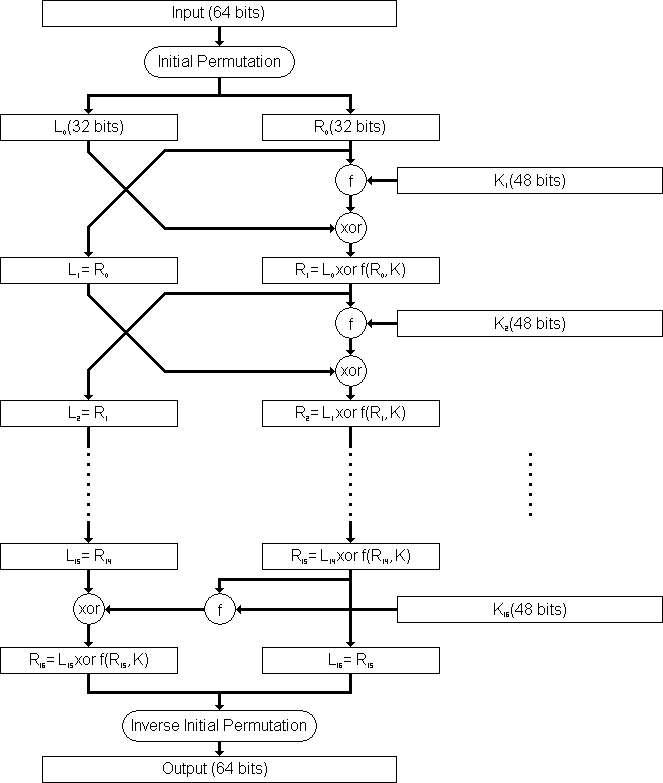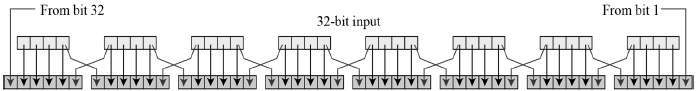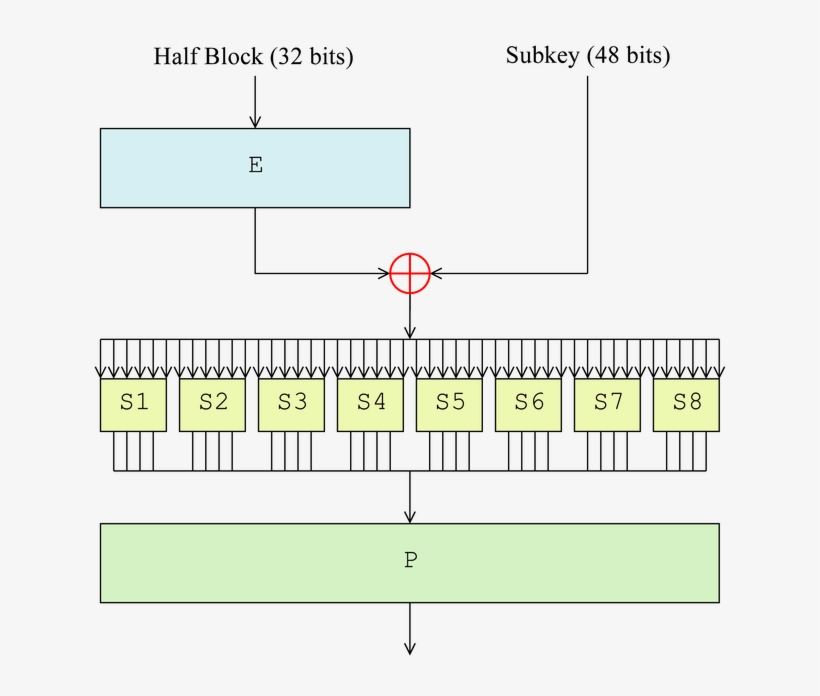## Data Encryption Standard

Data encryption standard is a symmetric-key block cipher published by National Institute of Standard and Technology(NIST).

### Symmetric-key block cipher

Let us first understand what this means. Every encryption algorithm uses a key to encrypt data and decrypt it. Since the key used for encryption and decryption is same in DES it is a symmetric-key cipher. It encrypts a 64-bit block at once so it is also a block cipher. Hence the name “symmetric-key block cipher”.

Now we are ready to dive into what happens to the data in DES:

The input data is passed through 16 similar rounds where the input is broken into two blocks(32-bits each) and the right block is passed through an encrypting function which gives 32-bit output. This output is then xor’ed with left block and the blocks obtained serve as input to the next round. Each round has a different 48-bit key which is generated from a 64-bit key using key-scheduling function.## Step1: Initial Permutation

The 64-bits input first goes through a standard permutation which is known publicly. It is available on the internet.

After this permutation, the rest of the steps are common to all 16 rounds.

## Step2: Expansion of input

The permuted input is now broken into two blocks of 32-bits each. The right block is always input of the encrypting function while the left block remains unchanged. So we have this 32-bits input and a 48-bits key. The first thing we do is expand the 32-bits input to 48 bits.## Step3: XOR input with key

The right block of input which was expanded to 48-bits is now xor’ed with the key. XOR means bit by bit addition modulo 2.

## Step3:S Boxes

This step makes use of S boxes which are non-linear mappings from 6-bits input to a 4-bits output. The xor’ed value (48 bit) from the previous step is divided into 8 blocks of 6-bits. The first block is fed to S1, second to S2 and so on. Each S box now maps the 6-bit entry to a 4-bit output, so the output of all S boxes form a 32-bits output.The inner configurations of S boxes is also available on the internet.

## Step4: Permutation again!

The output of S boxes, forming 32-bits, is again permuted to give 32-bits block according to another standard permutation table.The encrypting function

## Step5: XOR with left block

The final output obtained from the previous step is now xor’ed with the left block. This xor’ed value now serves as the right block for next round and the left block will be the right block of the previous round before operating the encrypting function.

## Step6: Last Permutation

After last round, the bits are permuted for one last time. This permutation is the opposite of initial permutation. It is chosen this way to make DES completely symmetric so that nothing new has to be done to decrypt ciphered data.

# Decryption

Decryption in DES is very easy. Since it is a symmetric cipher, all we need to do is put the encrypted text as input and use keys in opposite order. The output you will get will be the plain text.

## Security

• DES was published as an official Federal Information Processing Standard (FIPS) for the United States in 1977.
• It is no more a secure standard.
• Due to small length of key(56-bits), key can be found using brute force method.
• Differential cryptanalysis can also reduce the area of search for the possible key.
• In July 2017, a chosen-plaintext attack utilizing a rainbow table can recover the DES key for a single specific chosen plaintext 1122334455667788 in 25 seconds. A new rainbow table has to be calculated per plaintext.